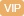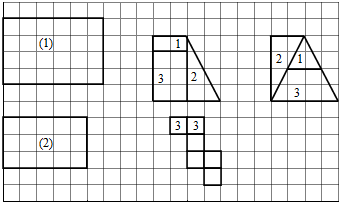| APP | 校园号 | 客服 客服热线：400-863-9889

400-863-9889（2019春•枣强县期末）直接写出得数：
 25×4= 360÷36= 0.3÷100= 8×9+8= 58-58= 1000×0.013 4.8+3.2= 101×28=
【考点】整数的乘法及应用整数的除法及应用小数乘法【专题】计算题；运算顺序及法则．

【分析】因为长与宽比是：，所以长和宽分是x厘米和2x厘米根据长方的面=长×宽即可求得；

【解答】解：设和宽分是x厘米和2x厘米
62=24

2×2=（厘）
1x16
3×=6厘米）
3x×2x24
S梯=（+）×42=12（平方厘米）
1÷（1+2+3=2（平方米）
x2=4
=1
×24（平方厘米）

3×13（米）
2×3（平方厘米）【点评】本题考查比用，按照总数及其比求每部分可；以及正体开图特、可能性的大小．

0/0